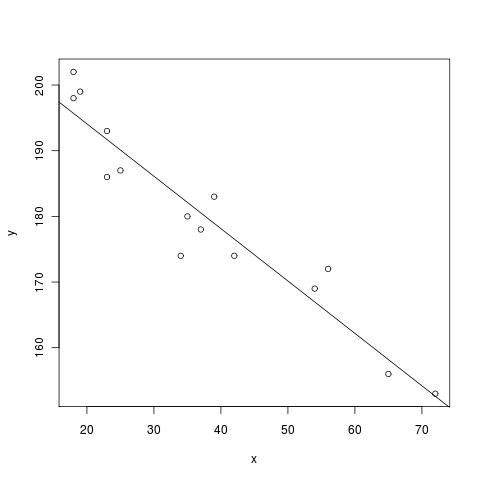# Sage Quickstart for Statistics¶

This Sage quickstart tutorial was developed for the MAA PREP Workshop “Sage: Using Open-Source Mathematics Software with Undergraduates” (funding provided by NSF DUE 0817071). It is licensed under the Creative Commons Attribution-ShareAlike 3.0 license (CC BY-SA).

Although Sage began as a project in algebra and geometry, it has many functions for statistics and finance. Particularly due to the R project being a component of Sage, we have very powerful statistical techniques at our disposal.

## Basic Descriptive Statistics¶

Some basic statistical functions are built right in.

sage: mean([1,2,3,5])
11/4

sage: std([1,2,2,4,5,6,8]) # The standard deviation
sqrt(19/3)


Once we get beyond such things, there are usually several ways to accomplish things – which can be complicated, but also powerful.

## Distributions¶

Let’s generate a random sample from a given type of continuous distribution using native Python random generators.

• We use the simplest method of generating random elements from a log normal distribution with (normal) mean 2 and $$\sigma=3$$.
• Notice that there is really no way around making some kind of loop.
sage: my_data=[lognormvariate(2,3) for i in range(10)]
sage: my_data # random
[13.189347821530054, 151.28229284782799, 0.071974845847761343, 202.62181449742425, 1.9677158880100207, 71.959830176932542, 21.054742855786007, 3.9235315623286406, 4129.9880239483346, 16.41063858663054]


We can check whether the mean of the log of the data is close to 2.

sage: mean([log(item) for item in my_data]) # random
3.0769518857697618


Here is an example using the Gnu scientific library under the hood.

• Let dist be the variable assigned to a continuous Gaussian/normal distribution with standard deviation of 3.
• Then we use the .get_random_element() method ten times, adding 2 each time so that the mean is equal to 2.
sage: dist=RealDistribution('gaussian',3)
sage: my_data=[dist.get_random_element()+2 for _ in range(10)]
sage: my_data # random
[3.18196848067, -2.70878671264, 0.445500746768, 0.579932075555, -1.32546445128, 0.985799587162, 4.96649083229, -1.78785287243, -3.05866866979, 5.90786474822]


For now, it’s a little annoying to get histograms of such things directly. Here, we get a larger sampling of this distribution and plot a histogram with 10 bins.

sage: my_data2 = [dist.get_random_element()+2 for _ in range(1000)]
sage: T = stats.TimeSeries(my_data)
sage: T.plot_histogram(normalize=False,bins=10)
Graphics object consisting of 10 graphics primitives


To access discrete distributions, we access another part of Sage which has statistics built in: Scipy.

• We have to import this module.
• We use binom_dist to denote the binomial distribution with 20 trials and 5% expected failure rate.
• The .pmf(x) method gives the probability of $$x$$ failures, which we then plot in a bar chart for $$x$$ from 0 to 20. (Don’t forget that range(21) means all integers from zero to twenty.)
sage: import scipy.stats
sage: binom_dist = scipy.stats.binom(20,.05)
sage: bar_chart([binom_dist.pmf(x) for x in range(21)])
Graphics object consisting of 1 graphics primitive


The bar_chart function performs some of the duties of histograms.

Scipy’s statistics can do other things too. Here, we find the median (as the fiftieth percentile) of an earlier data set. (We use a Python int to work around a bug in Numpy.)

sage: scipy.stats.scoreatpercentile(my_data, int(50)) # random
0.51271641116183286


The key thing to remember here is to look at the documentation!

• Particularly for Scipy, not everything in Sage is “wrapped” with an easy command, so you may have to do some experimentation.
• Improving this documentation would be a great way to get students involved.

## Using R from within Sage¶

There are several other pieces of Sage that have statistical capabilities, but by far the most important is the R project , which is the industry and academic standard for statistical analysis of all kinds.

There are several ways to access R.

• One of the easiest is to just put r() around things you want to make into statistical objects, and then …
• Use R commands via r.method() to pass them on to Sage for further processing.

The following example of the Kruskal-Wallis test comes directly from the examples in r.kruskal_test? in the notebook.

sage: x=r([2.9, 3.0, 2.5, 2.6, 3.2]) # normal subjects
sage: y=r([3.8, 2.7, 4.0, 2.4])      # with obstructive airway disease
sage: z=r([2.8, 3.4, 3.7, 2.2, 2.0]) # with asbestosis
sage: a = r([x,y,z]) # make a long R vector of all the data
sage: b = r.factor(5*+4*+5*) # create something for R to tell which subjects are which
sage: a; b # show them
 2.9 3.0 2.5 2.6 3.2 3.8 2.7 4.0 2.4 2.8 3.4 3.7 2.2 2.0
 1 1 1 1 1 2 2 2 2 3 3 3 3 3
Levels: 1 2 3

sage: r.kruskal_test(a,b) # do the KW test!
Kruskal-Wallis rank sum test

data:  sage17 and sage33
Kruskal-Wallis chi-squared = 0.7714, df = 2, p-value = 0.68


Looks like we can’t reject the null hypothesis here.

The best way to use R seriously is to simply ask each individual cell to evaluate completely in R, using a so-called “percent directive”. Here is a sample linear regression from John Verzani’s simpleR text. Notice that R also uses the # symbol to indicate comments.

sage: %r
....: x = c(18,23,25,35,65,54,34,56,72,19,23,42,18,39,37) # ages of individuals
....: y = c(202,186,187,180,156,169,174,172,153,199,193,174,198,183,178) # maximum heart rate of each one
....: png() # turn on plotting
....: plot(x,y) # make a plot
....: lm(y ~ x) # do the linear regression
....: abline(lm(y ~ x)) # plot the regression line
....: dev.off()     # turn off the device so it plots
Call:
lm(formula = y ~ x)

Coefficients:
(Intercept)            x
210.0485      -0.7977

null device
1To get a whole worksheet to evaluate in R (and be able to ignore the %), you could also drop down the r option in the menu close to the top which currently has sage in it.

(There is also yet another Python interface to R called the rpy2 interface, but we do not currently recommend its use with Sage.)